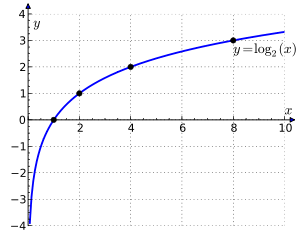# How Well Do You Know Logarithm Function?

10 Questions | Total Attempts: 126SettingsAre you a math geek? Do you love math? How versed are you in this topic? Take this quiz to find out what you know.

• 1.
Logarithmic functions are the inverses of exponential
• A.

Verses

• B.

Functions

• C.

Numbers

• D.

Figures

• 2.
The logarithm of a function is equal to the
• A.

Figures

• B.

Graph

• C.

Exponential equation

• D.

Equation

• 3.
A logarithmic function can be solved
• A.

With calculator

• B.

With numbers

• C.

Figurely

• D.

Graphically

• 4.
A logarithm is an
• A.

Inverse

• B.

Exponent

• C.

Independent figure

• D.

Inverse proportion

• 5.
The natural log uses the base
• A.

E

• B.

A

• C.

I

• D.

K

• 6.
A step function is a discontinuous
• A.

Order

• B.

Figure

• C.

Function

• D.

Number

• 7.
What is used to explore logarithmic functions?
• A.

Graph

• B.

Applet

• C.

Calculator

• D.

Table

• 8.
The logarithm is
• A.

Numeric

• B.

Transcendental

• C.

Graphic

• D.

Number

• 9.
The logarithm is a
• A.

Figure

• B.

Real number

• C.

Table

• D.

Graph

• 10.
The logarithm is useful in calculating
• A.

Figures

• B.

Base

• C.

Entropy

• D.

Power

Related TopicsBack to top